Latest Banking jobs   »   NIACL AO reasoning

# Reasoning Quiz For NIACL AO Prelims 2023-23rd August

Directions (1-5): The question given below is followed by two statements numbered I and II. You have to decide whether the data provided in the statements sufficient to answer the question.

Q1. There are six persons namely, M through R of different ages. Who is the oldest person?
Statements:
I. Q is older than M, who is older than O. R is younger than P.
Il. P is older than Q. N is not the oldest person.

(a) If the data in the statement I alone is sufficient to answer the question, while the data in statement II alone is not sufficient to answer the question.
(b) If the data in the statement II alone is sufficient to answer the question, while the data in statement I alone is not sufficient to answer the question.
(c) If the data either in the statement I alone or in statement II alone is sufficient to answer the question.
(d) If the data in both the statements I and II together are not sufficient to answer the question.
(e) If the data in both the statements I and II together are necessary to answer the question.

Q2. Six boxes, D, E, F, J, K and L are placed one above another but not necessarily in the same order, where the bottommost box is numbered as 1 and the box just above it is numbered as 2 and so on. Which box is placed at the topmost position?
Statements:
I. D is placed 2nd to the above of K, which is placed just above of E. Two boxes are placed between K and L.
II. J is placed above D. F is not placed at the even numbered position.

(a) If the data in the statement I alone is sufficient to answer the question, while the data in statement II alone is not sufficient to answer the question.
(b) If the data in the statement II alone is sufficient to answer the question, while the data in statement I alone is not sufficient to answer the question.
(c) If the data either in the statement I alone or in statement II alone is sufficient to answer the question.
(d) If the data in both the statements I and II together are not sufficient to answer the question.
(e) If the data in both the statements I and II together are necessary to answer the question.

Q3. Six persons (W, X, Y, Z, U and V) are sitting around a circular table facing towards the center of the table but not necessarily in the same order. Who sits 2nd to the right of W?
Statements:
I. V sits adjacent to U. U sits 2nd to the left of W. X sits 2nd to the left of V.
II. U sits 2nd to the right of Z. Y sits adjacent to W.

(a) If the data in the statement I alone is sufficient to answer the question, while the data in statement II alone is not sufficient to answer the question.
(b) If the data in the statement II alone is sufficient to answer the question, while the data in statement I alone is not sufficient to answer the question.
(c) If the data either in the statement I alone or in statement II alone is sufficient to answer the question.
(d) If the data in both the statements I and II together are not sufficient to answer the question.
(e) If the data in both the statements I and II together are necessary to answer the question.

Q4. Eight boxes A, B, C, D, Q, R, S and T are kept one above another but not necessarily in the same order. The bottommost box is numbered as 1 and the topmost box is numbered as 8. How many boxes are kept between Box A and Box R?
Statements:
I. Only three boxes are kept below Box B. Three boxes are kept between Box B and Box T. Box R is kept at one of the places above Box N.
II. Only three boxes are kept between Box Q and Box R. Only one box is kept between Box R and Box D.

(a) If the data in the statement I alone is sufficient to answer the question, while the data in statement II alone is not sufficient to answer the question.
(b) If the data in the statement II alone is sufficient to answer the question, while the data in statement I alone is not sufficient to answer the question.
(c) If the data either in the statement I alone or in statement II alone is sufficient to answer the question.
(d) If the data in both the statements I and II together are not sufficient to answer the question.
(e) If the data in both the statements I and II together are necessary to answer the question.

Q5. Eight persons J, K, L, M, N, G, H, and I are born on 1st in the different months from January to August but not necessarily in the same order. Who is the youngest among them?
Statements:
I. Two persons born between N and G, who was born immediately after I.
II. M was born before I but after H.

(a) If the data in the statement I alone is sufficient to answer the question, while the data in statement II alone is not sufficient to answer the question.
(b) If the data in the statement II alone is sufficient to answer the question, while the data in statement I alone is not sufficient to answer the question.
(c) If the data either in the statement I alone or in statement II alone is sufficient to answer the question.
(d) If the data in both the statements I and II together are not sufficient to answer the question.
(e) If the data in both the statements I and II together are necessary to answer the question.

Directions (6-8): Answer the questions based on the information given below.
Seven persons are sitting at some distance from each other. W is sitting 4m west to X. T is sitting 2m north to S, who is sitting 4m west to Q, who is sitting 1m north to V. W is sitting 2m north to Y. Y is sitting 8m west of T.

Q6. What is the direction of X with respect to Q?
(a) North-east
(b) South-east
(c) North
(d) North-west
(e) None of these

Q7. What is the shortest distance between W and V?
(a) 13m
(b) 15m
(c) 11m
(d) 9m
(e) 8m

Q8. What is the direction of S with respect to W?
(a) North-east
(b) South-east
(c) South-west
(d) North-west
(e) None of these

Directions (9-10): Answer the questions based on the information given below.
In a certain code,
A & B means A is 9m west of B.
A % B means A is 4m south of B.
A * B means A is 7m north of B.
A @ B means A is 5m east of B.

Q9. In expression ‘M&N%A%C&O*P*Q’, then Q is in which direction with respect to C?
(a) North-east
(b) South-east
(c) South-west
(d) North-west
(e) None of these

Q10. In expression ‘P&R&T@S%Q*L*M’, what is shortest distance between P and L?
(a) √178m
(b) √176m
(c) √185m
(d) √204m
(e) None of these

Solutions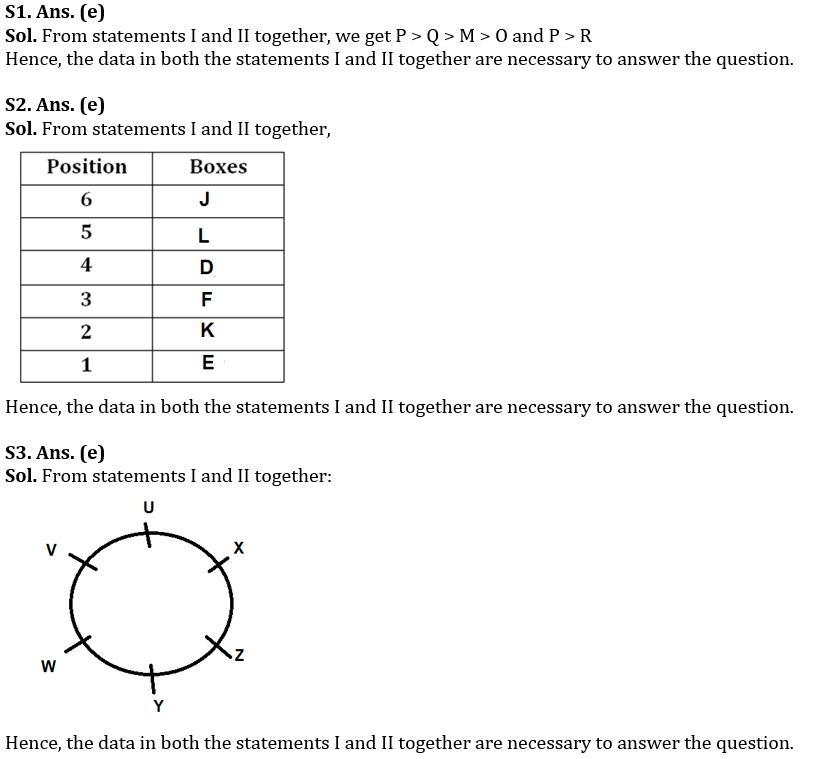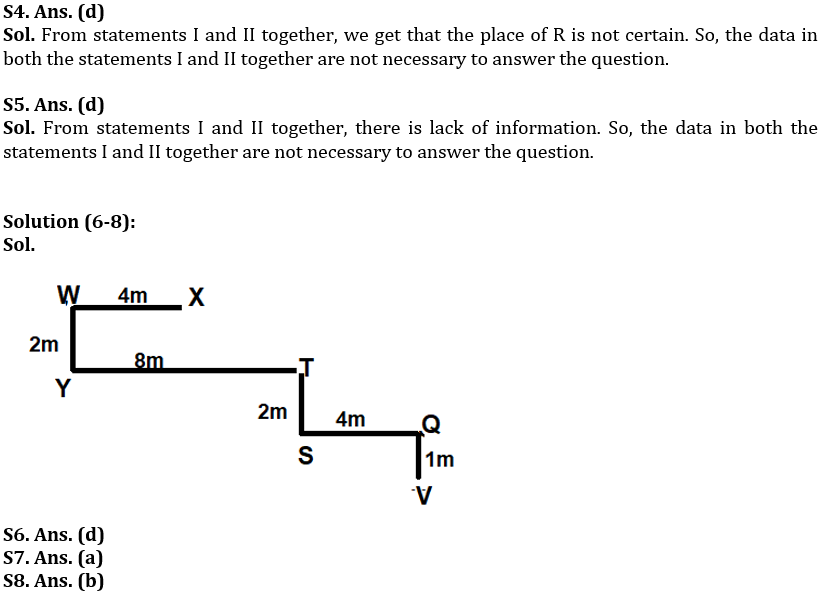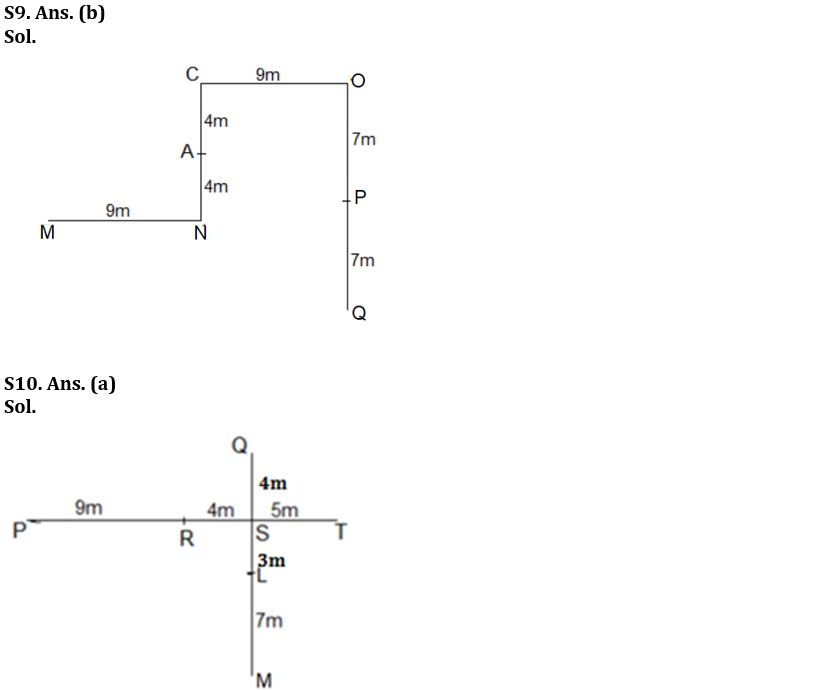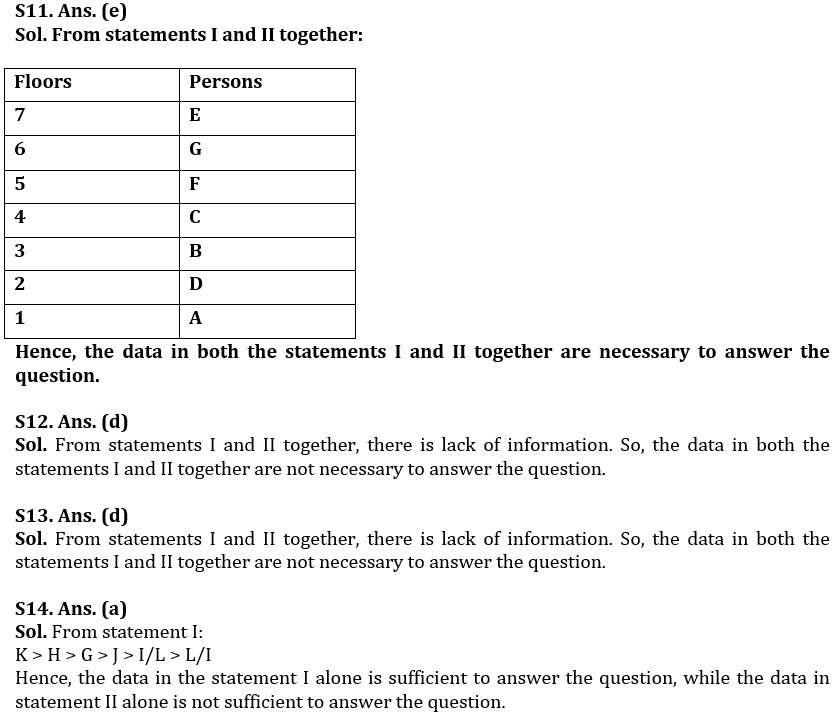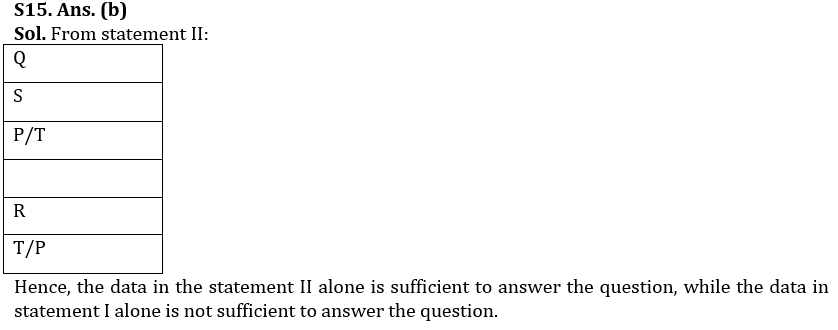## FAQs

### What are the subjects asked in NIACL AO Prelims exam 2023?

Subjects asked in NIACL AO Prelims are Reasoning Ability

#### Congratulations!Union Budget 2023-24: Free PDF